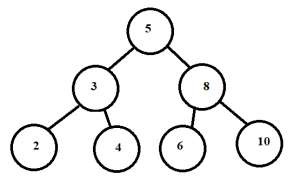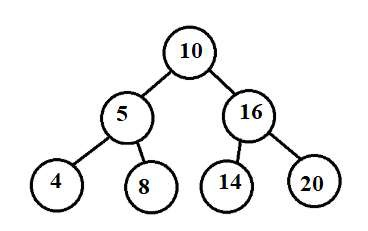# Count BST subtrees that lie in given range in C++

We are given a Binary search tree as an input. The goal is to find the count of subtrees in BST which have node values in between the range of start and end. If start is 5 and end is 50. Then count subtrees in BST whose all nodes have weights >=5 and <=50.

Input − tree given below − range [ 3-6 ]Output − Count of tree lying in range − 2

Explanation − For nodes 4 and 6 only. Their subtrees ( NULL ) lie between 3-6.

Input − tree given below: range [ 12-20 ]Output − Count of tree lying in range − 3

Explanation − For nodes 16, 14 and 20. Their subtrees lie between 12-20.

## Approach used in the below program is as follows

• Structure Btreenode is used to create a node of a tree, with info part as integer and self referencing left and right pointers to point to subtrees.

• Function Btreenode* insert(int data) is used to create a node with data as info and left and right pointers as NULL.

• Create a BST using insert function by calling it for a given structure. To add nodes in right to the root node ( root->right = insert(70); ) and to the left of root ( root->left = insert(30); ).

• Variables l and h are used to store minimum and maximum value of a range.

• Variable count stores the count of BST’s inside the tree that are in range between l and h. Initially 0.

• Function getBtreeCount(Btreenode* root, int low, int high, int* count) takes the root of BST, left and right boundaries of range and address of count as parameters and updates the value of count for each recursive call.

• For current root check if it is NULL, if yes return 1 as it is not part of the tree.

• For current nodes, check for all its left and right subtree node’s whether they lie in the given range. By recursive calls getBtreeCount(root->left, low, high, count); and getBtreeCount(root->right, low, high, count);

• If both subtrees are lying between the range and the current node is also lying in the range then the tree rooted at the current node is in range. Increment count. if (left && right && root->info >= low && root->info <= high) and ++*count; return 1.

• At the end count will have updated value as count of all subtrees.

• Print the result in count.

## Example

Live Demo

#include <bits/stdc++.h>
using namespace std;
// A BST node
struct Btreenode {
int info;
Btreenode *left, *right;
};
int getBtreeCount(Btreenode* root, int low, int high, int* count){
// Base case
if (root == NULL)
return 1;
int left = getBtreeCount(root->left, low, high, count);
int right = getBtreeCount(root->right, low, high, count);
if (left && right && root->info >= low && root->info <= high) {
++*count;
return 1;
}
return 0;
}
Btreenode* insert(int data){
Btreenode* temp = new Btreenode;
temp->info = data;
temp->left = temp->right = NULL;
return (temp);
}
int main(){
/* BST for input
50
/ \
30 70
/ \ / \
20 40 60 80 */
Btreenode* root = insert(50);
root->left = insert(30);
root->right = insert(70);
root->left->left = insert(20);
root->left->right= insert(40);
root->right->left = insert(60);
root->right->right = insert(80);
int l = 10;
int h = 50;
int count=0;
getBtreeCount(root, l, h, &count);
cout << "Count of subtrees lying in range: " <<count;
return 0;
}

## Output

Count of subtrees lying in range: 3